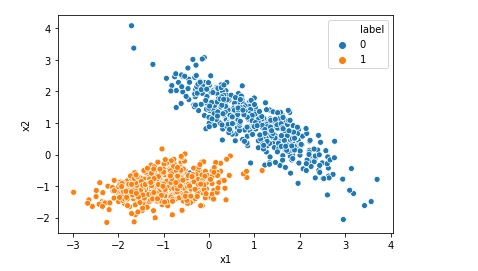# Implementing Logistic Regression in 10 lines in Python

Logistic Regression is one of the most popular Machine Learning algorithm used for the classification problems. It should be noted that though there is a regression word in the name of the algorithm Logistic Regression, it is used for classification problems. A use case of Logistic regression could be, based on the symptoms for a disease that a patient has Logistic … Continue reading Implementing Logistic Regression in 10 lines in Python